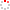IMR OpenIR
 A STABILIZED EQUAL-ORDER FINITE VOLUME METHOD FOR THE STOKES EQUATIONS Alternative Title A STABILIZED EQUAL-ORDER FINITE VOLUME METHOD FOR THE STOKES EQUATIONS Tian Wanfu1; Song Liqiu2; Li Yonghai3 2012 Source Publication JOURNAL OF COMPUTATIONAL MATHEMATICSISSN 0254-9409 Volume 30Issue:6Pages:615-628 Abstract We construct a new stabilized finite volume method on rectangular grids for the Stokes equations. The lowest equal-order conforming finite element pair (piecewise bilinear velocities and pressures) and piecewise constant test spaces for both the velocity and pressure are employed in this method. We show the stability of this method and prove first optimal rate of convergence for the velocity in the H-1 norm and the pressure in the L-2 norm. In addition, a second order optimal error estimate for the velocity in the L-2 norm is derived. Numerical experiments illustrating the theoretical results are included. Other Abstract We construct a new stabilized finite volume method on rectangular grids for the Stokes equations. The lowest equal-order conforming finite element pair (piecewise bilinear velocities and pressures) and piecewise constant test spaces for both the velocity and pressure are employed in this method. We show the stability of this method and prove first optimal rate of convergence for the velocity in the H~1 norm and the pressure in the L~2 norm. In addition, a second order optimal error estimate for the velocity in the L~2 norm is derived. Numerical experiments illustrating the theoretical results are included. Keyword GENERALIZED DIFFERENCE-METHODS ELEMENT METHODS INCOMPRESSIBLE-FLOW COVOLUME METHOD Stokes equations Equal-order finite element pair Finite volume method Error estimate Indexed By CSCD Language 英语 Funding Project [985 program of Jilin University] ; [National Natural Science Foundation of China] CSCD ID CSCD:4707995 Citation statistics Document Type 期刊论文 Identifier http://ir.imr.ac.cn/handle/321006/150262 Collection 中国科学院金属研究所 Affiliation 1.中国科学院金属研究所2.中山大学3.吉林大学 Recommended CitationGB/T 7714 Tian Wanfu,Song Liqiu,Li Yonghai. A STABILIZED EQUAL-ORDER FINITE VOLUME METHOD FOR THE STOKES EQUATIONS[J]. JOURNAL OF COMPUTATIONAL MATHEMATICS,2012,30(6):615-628. APA Tian Wanfu,Song Liqiu,&Li Yonghai.(2012).A STABILIZED EQUAL-ORDER FINITE VOLUME METHOD FOR THE STOKES EQUATIONS.JOURNAL OF COMPUTATIONAL MATHEMATICS,30(6),615-628. MLA Tian Wanfu,et al."A STABILIZED EQUAL-ORDER FINITE VOLUME METHOD FOR THE STOKES EQUATIONS".JOURNAL OF COMPUTATIONAL MATHEMATICS 30.6(2012):615-628.
 Files in This Item: There are no files associated with this item.
 Related Services Recommend this item Bookmark Usage statistics Export to Endnote Google Scholar Similar articles in Google Scholar [Tian Wanfu]'s Articles [Song Liqiu]'s Articles [Li Yonghai]'s Articles Baidu academic Similar articles in Baidu academic [Tian Wanfu]'s Articles [Song Liqiu]'s Articles [Li Yonghai]'s Articles Bing Scholar Similar articles in Bing Scholar [Tian Wanfu]'s Articles [Song Liqiu]'s Articles [Li Yonghai]'s Articles Terms of Use No data! Social Bookmark/Share